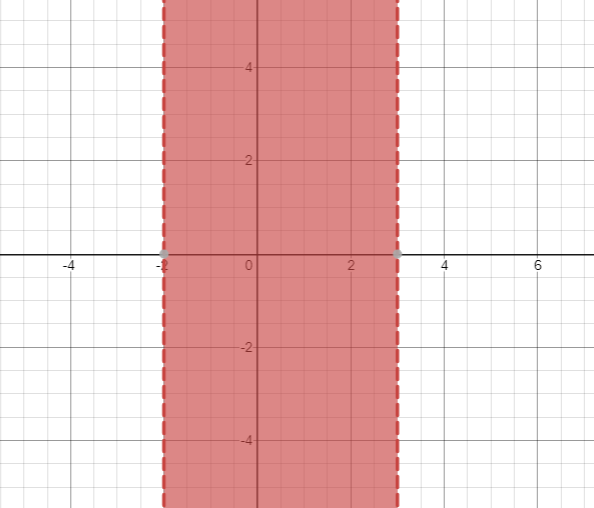# The solution of given nonlinear inequality equation and plot a graph for it.### Precalculus: Mathematics for Calcu...

6th Edition
Stewart + 5 others
Publisher: Cengage Learning
ISBN: 9780840068071### Precalculus: Mathematics for Calcu...

6th Edition
Stewart + 5 others
Publisher: Cengage Learning
ISBN: 9780840068071

#### Solutions

Chapter 1.7, Problem 35E
To determine

## The solution of given nonlinear inequality equation and plot a graph for it.

Expert Solution

2<x<3

### Explanation of Solution

Given:

Non-linear inequality equation

(x+2)(x3)<0

Calculation,

Non-linear inequality equation,

(x+2)(x3)<0(x+2)<0x>2or,(x3)<0x<3so,2<x<3

Now, graph of (x+2)(x3)<0Conclusion:

Hence, the values of x lies between the -2 and 3.

### Have a homework question?

Subscribe to bartleby learn! Ask subject matter experts 30 homework questions each month. Plus, you’ll have access to millions of step-by-step textbook answers!